Electron. J. Diff. Equ., Vol. 2010(2010), No. 33, pp. 1-11.

### Existence of multiple solutions for a p(x)-Laplace equation Duchao Liu

Abstract:
This article shows the existence of at least three nontrivial solutions to the quasilinear elliptic equation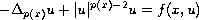in a smooth bounded domain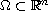, with the nonlinear boundary condition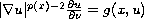or the Dirichlet boundary condition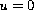on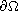. In addition, this paper proves that one solution is positive, one is negative, and the last one is a sign-changing solution. The method used here is based on Nehari results, on three sub-manifolds of the space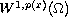.

Submitted September 26, 2008. Published March 3, 2010.
Math Subject Classifications: 35B38, 35D05, 35J20.
Key Words: Critical points; p(x)-Laplacian; integral functionals; generalized Lebesgue-Sobolev spaces.

Show me the PDF file (242 KB), TEX file, and other files for this article.Duchao Liu School of Mathematics and Statistics Lanzhou University, Lanzhou 730000, China email: liudch06@lzu.cn